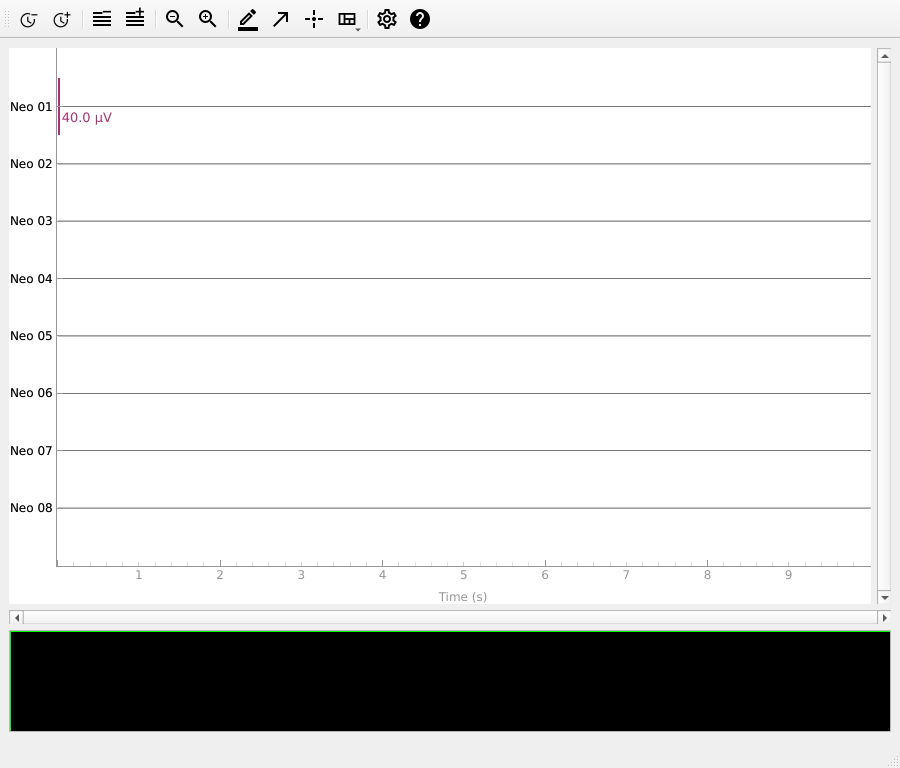# How to use data in neural ensemble (NEO) format#

This example shows how to create an MNE-Python `Raw` object from data in the neural ensemble format. For general information on creating MNE-Python’s data objects from NumPy arrays, see Creating MNE-Python data structures from scratch.

```import neo
import mne
```

This example uses NEO’s `ExampleIO` object for creating fake data. The data will be all zeros, so the plot won’t be very interesting, but it should demonstrate the steps to using NEO data. For actual data and different file formats, consult the NEO documentation.

```reader = neo.io.ExampleIO('fakedata.nof')
segment = block.segments         # get data from first (and only) segment
signals = segment.analogsignals  # get first (multichannel) signal

data = signals.rescale('V').magnitude.T
sfreq = signals.sampling_rate.magnitude
ch_names = [f'Neo {(idx + 1):02}' for idx in range(signals.shape)]
ch_types = ['eeg'] * len(ch_names)  # if not specified, type 'misc' is assumed

info = mne.create_info(ch_names=ch_names, ch_types=ch_types, sfreq=sfreq)
raw = mne.io.RawArray(data, info)
raw.plot(show_scrollbars=False)
``````Creating RawArray with float64 data, n_channels=8, n_times=100000
Range : 0 ... 99999 =      0.000 ...    10.000 secs